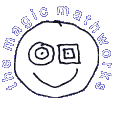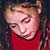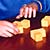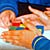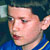Magic Mathworks Travelling CircusExperiencing Mathematics through Sight, Sound, Touch and Movement# Explorations

‘Explorations’ are topics for students to investigate on their own. Though little hands-on work is  included, we take advantage of the software package GeoGebra in several cases.

In ‘Tiling transformations’ we perform operations to change one uniform tiling into another.

Tiling transformations

In ‘Shape counts in a tiling’ we find the ratio of the numbers of the different tiles present in a uniform tiling.

Shape counts in a tiling

‘Number City rows’ concerns the station ‘Number City’ under the topic heading ‘Multiplication’.

Number city rows

‘Figurate numbers’ summarises and extends the results of the masterclass of the same name.

Figurate numbers

‘Dissection algebra’ is about finding area ratios without calculation, namely by dissection.

Dissection algebra

‘Shape & size’ shows how thirteen heuristic principles help derive and check formulas for the areas and volumes of standard shapes, and the positions of the centroid in them. Calculus is avoided, though students familiar with integral calculus can exercise their skills in finding alternative derivations.

Shape & size

The Young Geometer’s Casebook (TYGCB) contains material to train students for the geometry problems in maths contests. Open ‘TYGCB 0’, the contents file, and choose a topic of interest.

The following is an e-book (.pdf file) in which each chapter explores a particular number shape (triangle, square, …). All relations are expressed in a ‘figurate’ algebra, (which may always be translated into ordinary algebra). It is a visual approach to elementary number theory.

Figurate Identities

Interdissectible tilings

In the following file we tackle the problem of constructing a regular polygon of n sides with the same area as one with 2n sides.

n-gon = 2n-gon

The subject of the next file is again dissections, but those forming sequences. Three types are distinguished.

Dissection sequences

The next file describes deriving tilings by an ‘expand-&-fill’ procedure.

Infill tilings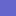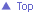The Magic Mathworks Travelling Circus